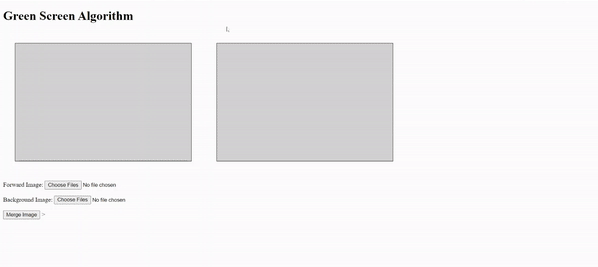# Implement Green Screen Algorithm using JavaScript

• Difficulty Level : Expert
• Last Updated : 12 Jan, 2021

The Green Screen Algorithm (also known as chromakey algorithm) is used to change the background image which is green color and replace it with any effect or other image. Basically what we are doing is, we are replacing all the green pixels in the forward image with the corresponding pixels from the background image. Also, we have to remember of the size of the output image which should be same as forward image. Then the pixels from the forward image are copied into the new image. The corresponding pixels of background image get copied instead of green pixels.

Before implementing the following code, do not forget to mention the below source file in your HTML code:

<script src=”https://www.dukelearntoprogram.com/course1/common/js/image/SimpleImage.js”></script>

Following is the JavaScript code to implement this algorithm. You have to write HTML Code yourself to implement it:

Example:

## HTML

 ` ` `<``html``> ` ` `  `<``head``> ` `    ``<``script` `src``= ` `"https://www.dukelearntoprogram.com/course1/common/js/image/SimpleImage.js"``> ` `    `` ` `     `  `    ``<``style``> ` `        ``canvas { ` `            ``background: lightgray; ` `            ``border: 1px solid black; ` `            ``width: 450px; ` `            ``height: 300px; ` `            ``margin: 30px; ` `        ``} ` `    `` ` ` ` ` `  `<``body``> ` ` `  `    ``<``h1``>Green Screen Algorithm ` ` `  `    ``<``canvas` `id``=``"pic1"``>  ` `    ``<``canvas` `id``=``"pic2"``> ` `    ``<``br` `/> ` ` `  `     `  `<``p``> ` `        ``Forward Image: <``input` `type``=``"file"`  `        ``id``=``"fgFile"` `multiple``=``"false"`  `        ``onChange``=``"frontimg()"``> ` `    `` ` ` `  `     `  `     `  `<``p``> ` `        ``Background Image: <``input` `type``=``"file"`  `        ``id``=``"bgFile"` `multiple``=``"false"`  `        ``onChange``=``"backimg()"``> ` `    `` ` ` `  `     `  `    ``<``input` `type``=``"button"` `value``=``"Merge Image"` `        ``onClick``=``"merge()"``> ` ` `  `    ``<``script` `type``=``"text/javascript"``> ` `        ``var imgFG = null; ` `        ``var imgBG = null; ` ` `  `        ``// This function takes forward image as input ` `        ``function frontimg() { ` `            ``var fileInput = document.getElementById("fgFile"); ` `            ``var canvas = document.getElementById("pic1"); ` `            ``imgFG = new SimpleImage(fileInput); ` `            ``imgFG.drawTo(canvas); ` `        ``} ` ` `  `        ``// This function takes background image as output ` `        ``function backimg() { ` `            ``var fileInput = document.getElementById("bgFile"); ` `            ``var canvas = document.getElementById("pic2"); ` `            ``imgBG = new SimpleImage(fileInput); ` `            ``imgBG.drawTo(canvas); ` `        ``} ` ` `  `        ``// This function merges both the image and ` `        ``// produces the resultant image as output ` `        ``// Implementation of Green Screen Algorithm ` `        ``function merge() { ` `            ``clear(); ` `            ``var pic1 = document.getElementById("pic1"); ` ` `  `            ``var outputImage = new SimpleImage( ` `                    ``imgFG.width, imgFG.height); ` ` `  `            ``for (var pixel of imgFG.values()) { ` `                ``if (pixel.getGreen() > pixel.getRed()  ` `                                ``+ pixel.getBlue()) { ` `                    ``var x = pixel.getX(); ` `                    ``var y = pixel.getY(); ` `                    ``var newPixel = imgBG.getPixel(x, y); ` `                    ``outputImage.setPixel(x, y, newPixel); ` `                ``} ` `                ``else { ` `                    ``outputImage.setPixel(pixel.getX(), ` `                            ``pixel.getY(), pixel); ` `                ``} ` `            ``} ` `            ``outputImage.drawTo(pic1); ` `        ``} ` ` `  `        ``// This function clears the previous  ` `        ``// fetched input and output. ` `        ``function clear() { ` `            ``var pic1 = document.getElementById("pic1"); ` `            ``var pic2 = document.getElementById("pic2"); ` `            ``var context = pic1.getContext("2d"); ` `            ``context.clearRect(0, 0, pic1.width, pic1.height); ` `            ``context = pic2.getContext("2d"); ` `            ``context.clearRect(0, 0, pic2.width, pic2.height); ` `        ``} ` `    ``> ` ` ` ` `  ``

Output:This algorithms take two images as input. One is forward image which contains green color in background, and other is background image which is to be replaced in place of green background.

After taking both images as input, the following code merges both the image and hence backward images replaces the green background of the forward image. So, above was the code to implement Green Screen Algorithm.

My Personal Notes arrow_drop_up
Recommended Articles
Page :